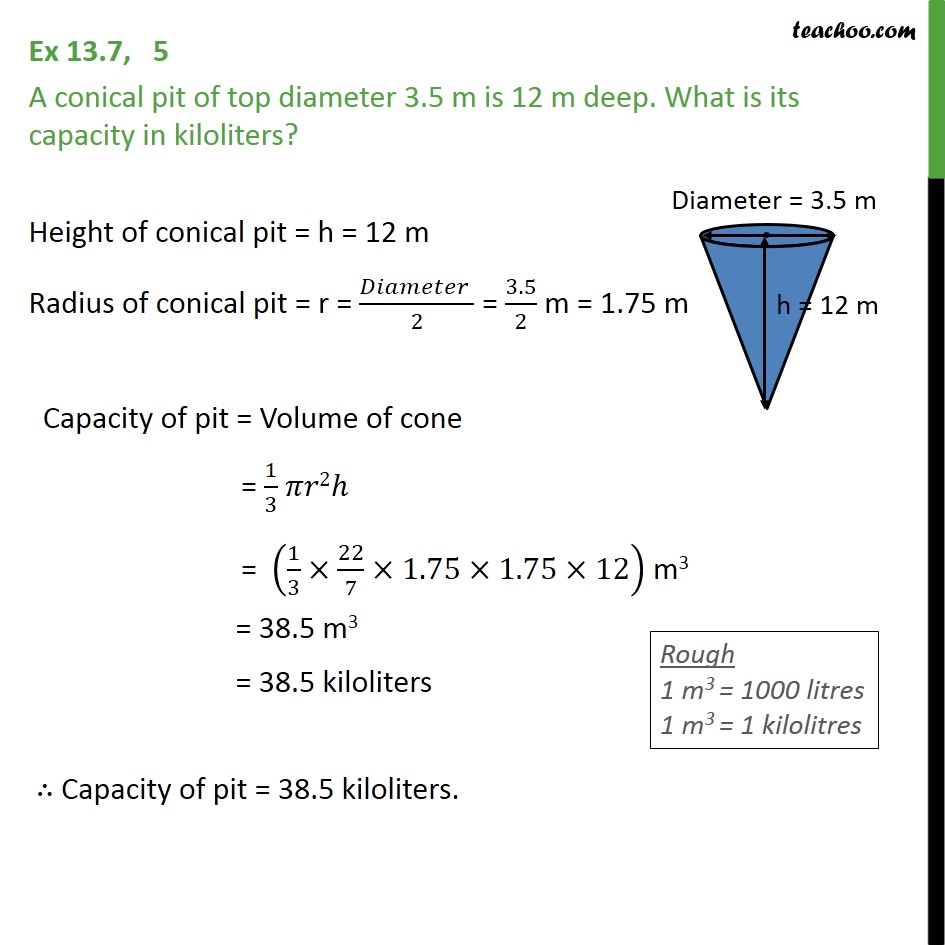Ex 11.3

Chapter 11 Class 9 Surface Areas and Volumes
Serial order wiseLearn in your speed, with individual attention - Teachoo Maths 1-on-1 Class

### Transcript

Ex 11.3, 5 A conical pit of top diameter 3.5 m is 12 m deep. What is its capacity in kiloliters? Height of conical pit = h = 12 m Radius of conical pit = r = ( )/2 = 3.5/2 m = 1.75 m Capacity of pit = Volume of cone = 1/3 2 = (1/3 22/7 1.75 1.75 12) m3 = 38.5 m3 = 38.5 kiloliters Capacity of pit = 38.5 kiloliters.